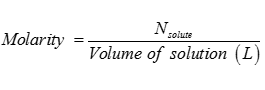# Chemistry Solutions - Online Test

Q1. Example of solution of liquid in gas is
Explaination / Solution:

Solute is water (liquid) and solvent is gas( air).

Q2. Molality of a solution relates the
Explaination / Solution:

Molality is number of moles of solute dissolved in per Kg of solvent.

Q3. Which law shows relation between pressure and solubility of a gas in solvent
Explaination / Solution:

Henry’s Law states that as the partial pressure of gas increases over liquid solubility of gas also increases.

Q4. For ideal solution the enthalpy of mixing of the pure components to form the solution is
Explaination / Solution:

For ideal solution there is no evolution or absorption of heat on mixing.

Q5. For ideal solution the volume of mixing of the pure components to form the solution is
Explaination / Solution:

For ideal solution there is no increase or decrease in volume on mixing.

Q6. Molarity of the solution is
Explaination / Solution:Q7. Raoult’s law states that for a solution of volatile liquids the partial pressure of each component in the solution is
Explaination / Solution:

Raoult’s law states that for a solution of volatile liquids the partial pressure of each component in the solution is directly proportional to mole fraction of component.

Q8. Minimum boiling azeotrope is form by the solution which show
Explaination / Solution:

Positively deviated non ideal solution forms Minimum boiling Point azeotropes.

Q9. Which of the following is a colligative property?
Explaination / Solution:

Colligative property is dependent on no. of moles of solute. (Л=cRT)

Q10. Cryoscopic constant of a liquid is
Explaination / Solution:

ΔTf= Kfm.  When m(molality )= 1m then kf= ΔTf